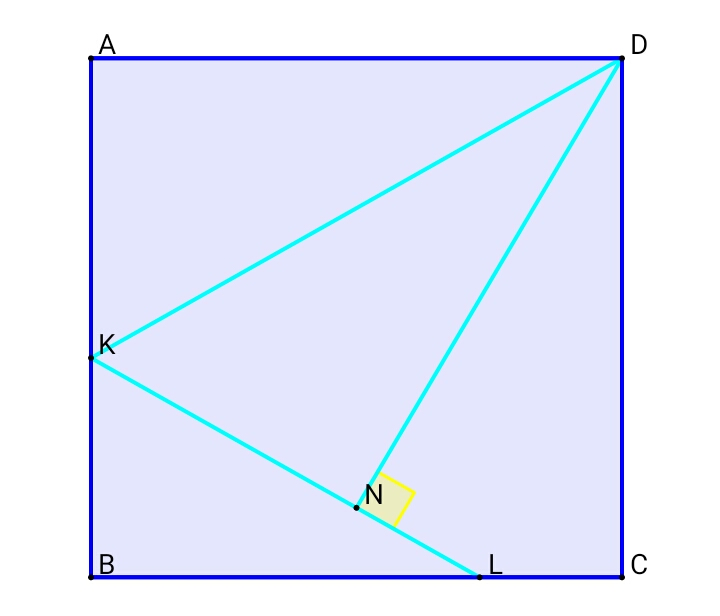# Algebra in Geometry!

Geometry Level 3$\angle KDN = \angle KDA$. Length of $KN =6\text{ cm}$ and length of $NL=4\text{ cm}$. $ABCD$ is a square.Find the length of $CD$ (in $\text{cm}$).

Try another problem on my set! Let's Practice

×